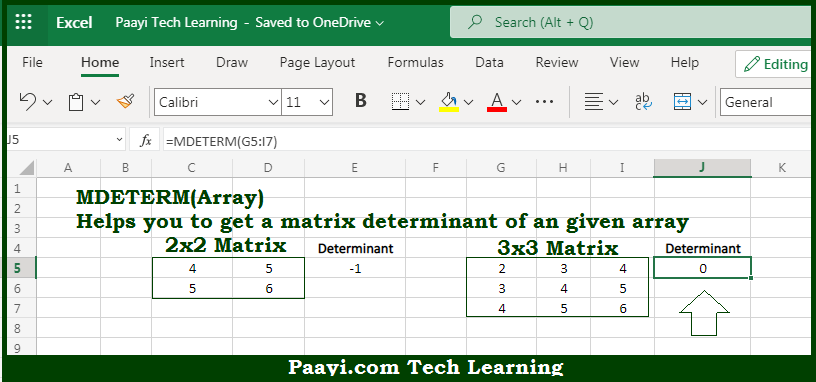# Learn How to Use Microsoft Excel MDETERM Function

Written by | 0 Comments | 442 Views

In this article, you will learn how to use the Microsoft Excel MDETERM function and its prime function in Microsoft Excel. You will also get to know the Microsoft Excel MDETERM function return value and syntax with the help of some examples.

Microsoft Excel MDETERM Function

The main function of Microsoft Excel MDETERM function is to get the matrix determinant of the given array. That implies, with the help of MDETERM function you can able to return the matrix determinant of the given array. It should be noted that the input array must contain numbers only and should be a square matrix, with equal rows and columns. The result will be the number representing the matrix determinant. In case there is text or blanks in the array, it will return the #VALUE! error. Also if there is not an equal number of rows and columns, the return value will be #VALUE!. So, with the help of MDETERM function, you can able to get the matrix determinant of the given array.

Return Value of MDETERM Function

The return value will be the number representing the matrix determinant.

Syntax of MDETERM Function

=MDETERM(array)

Where the arguments:

• array: This is the square array of numbers only.

How to Use Microsoft Excel MDETERM Function?So we know that Microsoft Excel MDETERM function you can able to get the matrix determinant of the given array. That implies, with the help of MDETERM function you can able to return the matrix determinant of the given array. It should be noted that the input array must contain numbers only and should be a square matrix, with equal rows and columns and no blanks. In case there is text or blanks in the array, it will return the #VALUE! error. Also if there is not an equal number of rows and columns, the return value will be #VALUE!. The result will be the number representing the matrix determinant. So, with the help of MDETERM function, you can able to get the matrix determinant of the given array.